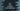# C++ class destructor explanation with examples## C++ destructor:

In this post, we will learn about destructors in C++ with examples. Destructors are special functions in C++ objects those are called when the object is destroyed. These are called automatically.

Constructors are called when the class objects are created and destructors are called when these are destroyed. Destructors are mostly used to free all resources that might be held by the class objects. This is required to avoid memory leaks.

### How the destructors are defined:

There is only one destructor defined in a class. The name of the destructor is the same as its class name prefixed with a tilde(~) symbol.

So, it is similar to the constructor with a tilde prefix.

Let’s learn how it works with examples.

### Example of C++ destructor:

Let’s consider the following example:

``````#include <iostream>
using namespace std;

class Student{
public:
Student()
{
cout<<"Constructor called"<<endl;
}
~Student(){
cout<<"Destructor called"<<endl;
}
};

int main()
{
Student s;
}``````

In this program, we created a class Student with a constructor and a destructor.

• Student() is the constructor, which is called when the class object is created.
• ~Student() is the destructor, which is called when the class object is destroyed.

If you run this program, it will print:

``````Constructor called
Destructor called``````

The program is creating a Student object and it stops. So, it calls the constructor and then calls the destructor.

### Example of multiple objects:

Let’s create multiple objects to check how constructors and destructors are called:

``````#include <iostream>
using namespace std;

class Student{
public:
Student()
{
cout<<"Constructor called"<<endl;
}
~Student(){
cout<<"Destructor called"<<endl;
}
};

int main()
{
Student s1, s2, s3, s4;
}``````

It is creating four Student objects. It will call the constructors of these objects sequentially and then call the destructors sequentially.

``````Constructor called
Constructor called
Constructor called
Constructor called
Destructor called
Destructor called
Destructor called
Destructor called``````

### Can we call a destructor explicitly:

Yes, we can call a destructor explicitly. We can call it similar to any other function call.

``````#include <iostream>
using namespace std;

class Student{
public:
Student()
{
cout<<"Constructor called"<<endl;
}
~Student(){
cout<<"Destructor called"<<endl;
}
};

int main()
{
Student s1;
s1.~Student();
}``````

In this example, we called the destructor explicitly, s1.~Student(). It will invoke the destructor two times, when we invoked it and when the object is destroyed.

So, it will print the message mentioned in the destructor twice:

``````Constructor called
Destructor called
Destructor called``````